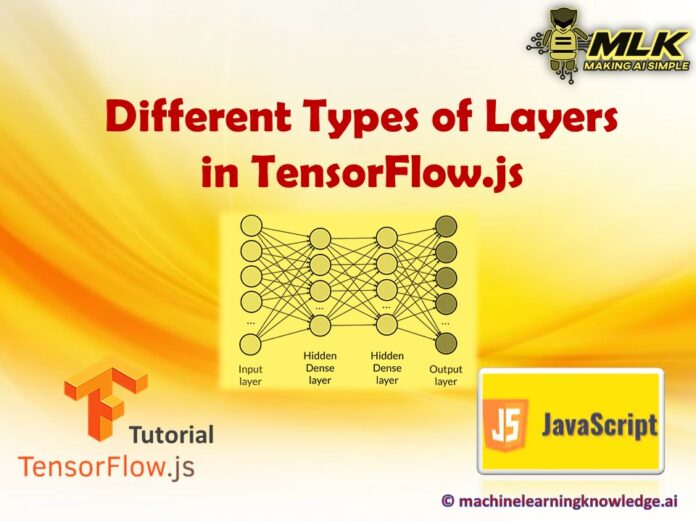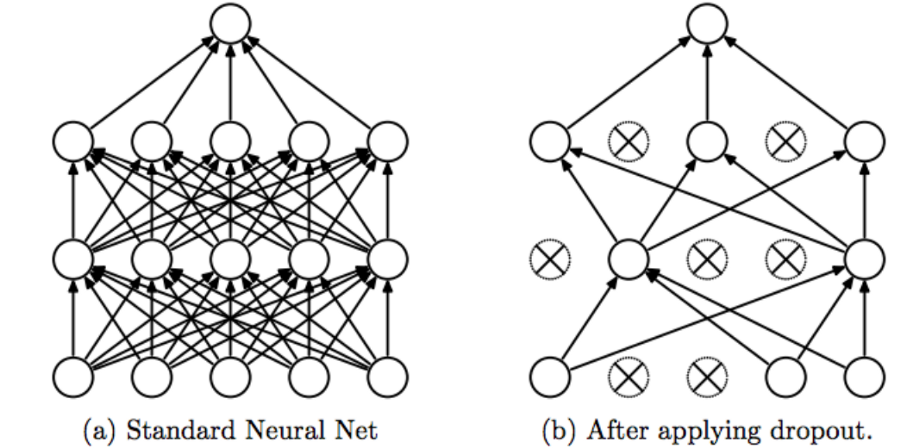# Different Types of Layers in Tensorflow.js## Introduction

Tensorflow.js is the most popular deep learning framework for javascript that can let you work with neural networks either on node.js or on the web browser. There are many types of layers in Tensorflow.js that help as the building blocks for creating various elements of simple to advanced neural networks. In this tutorial, we will take a high-level view of these Tensorflow.js layers that will be helpful for beginners.

## Types of Layers in Tensorflow.js – API vs Custom

Tensorflow.js is built on top of a math API (ops API) coupled with a Keras-like layers API. Which lets one build, save, import, export models. Additionally, it provides access to numerous layers which lets one harness the power of neural networks at ease.

### i) Layers API

Similar to the Keras API, the layers API of Tensiorflow.js allows us to create neural network models like blocks of legos. In order to create a model with the layers API the ‘add()’ function is called on either a ‘tf.Sequential’ object or ‘tf.LayersModel’ object.

#### Example

Prerequisite: Add this script tag to enable your browser to run tensorflow.js.

``<script src="https://cdn.jsdelivr.net/npm/@tensorflow/[email protected]/dist/tf.min.js"></script>``

Here we are defining a ‘tf.Sequential’ object name ‘model’ and using the ‘add()’ function to add a dense layer to the model. After that, the model needs to be compiled for use using yet another function from the layers API called ‘tf.compile()’.

```const model = tf.sequential()
model.compile({loss:"meanSquaredError",optimizer:"sgd"});
console.log(model.summary())```

Output:

```Layer (type)   Output shape     Param #
dense_Dense1 (Dense) [null,1]     2
"Total params: 2"
"Trainable params: 2"
"Non-trainable params: 0"```

### ii) Custom Layers

When doing research work on neural networks, you may need to do certain customizations for your requirement and this is where Custom Layer becomes useful in Tensorflow.js.

#### Example

For example, we may require intermediate layer results or transitional pre-processing and so for that function, custom layers are built using the OOP format.

``````class SquaredSumLayer extends tf.layers.Layer {
constructor() {
super({});
}
// In this case, the output is a scalar.
computeOutputShape(inputShape) { return []; }

// call() is where we do the computation.
call(input, kwargs) { return input.square().sum();}

// Every layer needs a unique name.
getClassName() { return 'SquaredSum'; }
}
const t = tf.tensor([-2, 1, 0, 5]);
const o = new SquaredSumLayer().apply(t);
o.print();``````

Output:

`30`

## Common Layers in Tensorflow.js

### i) Dense Layers

The most basic layer in Tensorflow.js for building neural network architectures is dense layers. It consists of fully connected layers i.e. each neuron is connected to every other neuron in the preceding or succeeding layer. It does the basic operation of applying the activation function to the dot product of input and kernel value.

`output = activation(dot(input, kernel) + bias)`

#### Example

```tf.layers.dense(arguments)
const Arguments= {
units: Positive_Integer,     # Output dimensions
inputShape: Shape_Array,     # Only defined in case of first layer of model
activation:Activation        # Type of activation to be used
}
//Example
tf.layers.dense({units:128,inputShape:})```

### ii) Convolutional Layers

Convolutional layers are the building blocks of convolutional neural networks. In layman’s terms, they apply kxk size filters to input in order to condense them into important features present in the input. For example, if this operation is applied to an image, the image size will decrease because all the information is being pulled together closer.

#### a) Conv1D

When a convolutional operation is performed using a single-dimensional filter it is known as temporal convolution and a ‘Conv1d’ layer is used to perform this operation.

```tf.layers.conv1d(Arguments)
const Arguments= {
filters: Positive_Integer,  # Output dimensions
inputShape: Shape_Array,    # Only defined in case of first layer of model
activation: Activation,     # Type of activation to be used
strides: Positive_Integer,  # Stride value refers to the movement of the kernel
}
// Example
tf.layers.conv1d({units:64,inputShape:})```

#### b) Conv2D

This layer creates a 2D kernel, which is convolved with the input and produces a smaller feature vector. Since these layers aren’t fully connected they are less costly and more accurate since they can help move faster toward converging since only selected neurons are fired at once.

```tf.layers.conv2d(Arguments)
const Arguments= {
filters: Positive_Integer, # Output dimensions
inputShape: Shape_Array,   # Only defined in case of first layer of model
activation: Activation,    # Type of activation to be used
strides: Positive_Integer, # Stride value refers to the movement of the kernel
}
// Example
tf.layers.conv2d({units:32,inputShape:})```

### iii) Normalization Layers

In order to bring the variations in data to a common scale, the normalization layer is used. For example, if a dataset contains the average age and the population of a city along with other features, the age feature will range from 0 to 90 but the population feature could range in millions. Thus normalization is required to standardize the data. In the case of neural networks, it is done by normalizing weights again and again with a predefined flag (batch or a layer).

#### a) Batch normalization

We know that a neural network takes input in batches so instead of normalizing whole data, the data is normalized according to small batches. This process helps in accelerated learning and shorter training times.

Example:

`tf.layers.batchNormalization()`

#### b) Layer Normalization

Applying the normalization process on the intermediate layers instead of the inputs refers to a process called layer normalization.

Example:

`tf.layers.layerNormalization()`

### iv) Flatten layer

Flatten layers are dimensionality-controlling layers. They reduce the no of dimensions in the input to a single dimension i.e. They convert multi-dimensional feature maps into a single vector. For example, a 32 by 32 image would become an array of 1024 elements

Example

`tf.layers.flatten();`

### v) Dropout LayerSrivastava, Nitish, et al. ”Dropout: a simple way to prevent neural networks from overfitting”, JMLR 2014

Dropout layers are common ways to regularize a neural network. In simple words during the training process, neurons are randomly fired or either switched off. It is proven research that dropouts can improve the generalization of the deep learning model.

Example

```tf.layers.dropout(Arguments)
const Arguments= {
rate: Positive_Integer,  # A float value between 0 & 1, that determines the no of values to be dropped
inputShape: Shape_Array, # Only defined in case of first layer of model
}```

### vi) Pooling Layers

Pooling layers are also called down-sampling layers because they greatly under-size the feature vector. In layman’s terms, it takes the features identified in a picture and focuses only on the important ones so that, only they are included in the next feature vector and everything else is discarded.

#### a) Average Pooling

Average pooling refers to the process of selecting the average value from each feature vector patch to be included in the next smaller feature map. Tensorflow.js provides 1,2 and 3-dimensional average pooling.

```tf.layers.averagePooling1d({
strides: positive_integer
poolSize: positive_integer
});```

Other layers

```tf.layers.averagePooling2d()
tf.layers.averagePooling3d()```

#### b) Max Pooling

Choosing the max value from each feature vector patch. This highlights the most important features, unlike the average value in the case of average pooling. It can be applied multiple times in a model in order to achieve a quick-fit model.

```tf.layers.maxPooling1d({
strides: positive_integer
poolSize: positive_integer
});```

Other variations:

```tf.layers.maxPooling2d();
tf.layers.maxPooling3d();```

#### c) Global pooling

In the case of global pooling instead of down-sampling patches of the feature map, the entire feature map is down-sampled to a single value. They are used in place of dense layers at the end of a model. The output of the global pooling layer is a single number which tells us that all the feature maps have been congealed into a single value.

Example

```const args = {
strides: positive_integer
poolSize: positive_integer
};
tf.layers.globalAveragePooling1d(args)```

Other variations

```tf.layers.globalMaxPooling1d(args)
tf.layers.globalAveragePooling2d(args)
tf.layers.globalMaxPooling2d(args)```

### vii) Recurrent Layers

RNN is a kind of artificial neural network designed to understand the sequential characteristics of data and predict the resulting patterns. It usually used in NLP and time series problems

#### a) LSTM

Long short term memory layers have an advantage over standard feed-forward neural networks and RNN in some ways. Due to their property of remembering selective memory patterns for long durations of your time. Information flow is carried over time, this way, LSTMs will remember or forget selective things. LSTMs build tiny modifications to the knowledge by multiplications and additions.
```const lstm = tf.layers.lstm({units: Positive_integer, returnSequences: Boolean});
units: No. of units in the layer
returnSequences: whether to return the last word or the whole sequence
// Example
tf.layers.lstm({units: 8, returnSequences: true}```

#### b) GRU

Most recurrent neural networks face the vanishing gradient problem i.e. For deep recurrent networks as the gradient progresses it becomes smaller and smaller to the point where the changes made are insignificant and the model ceases to improve. For this reason, gated recurrent units are used that possess the ability to reset when the gradient becomes too small.

```const lstm = tf.layers.gru({units: Positive_integer, returnSequences: Boolean});
units: No. of units in the layer
returnSequences: whether to return the last word or the whole sequence
// Example
tf.layers.gru({units: 8, returnSequences: true}```